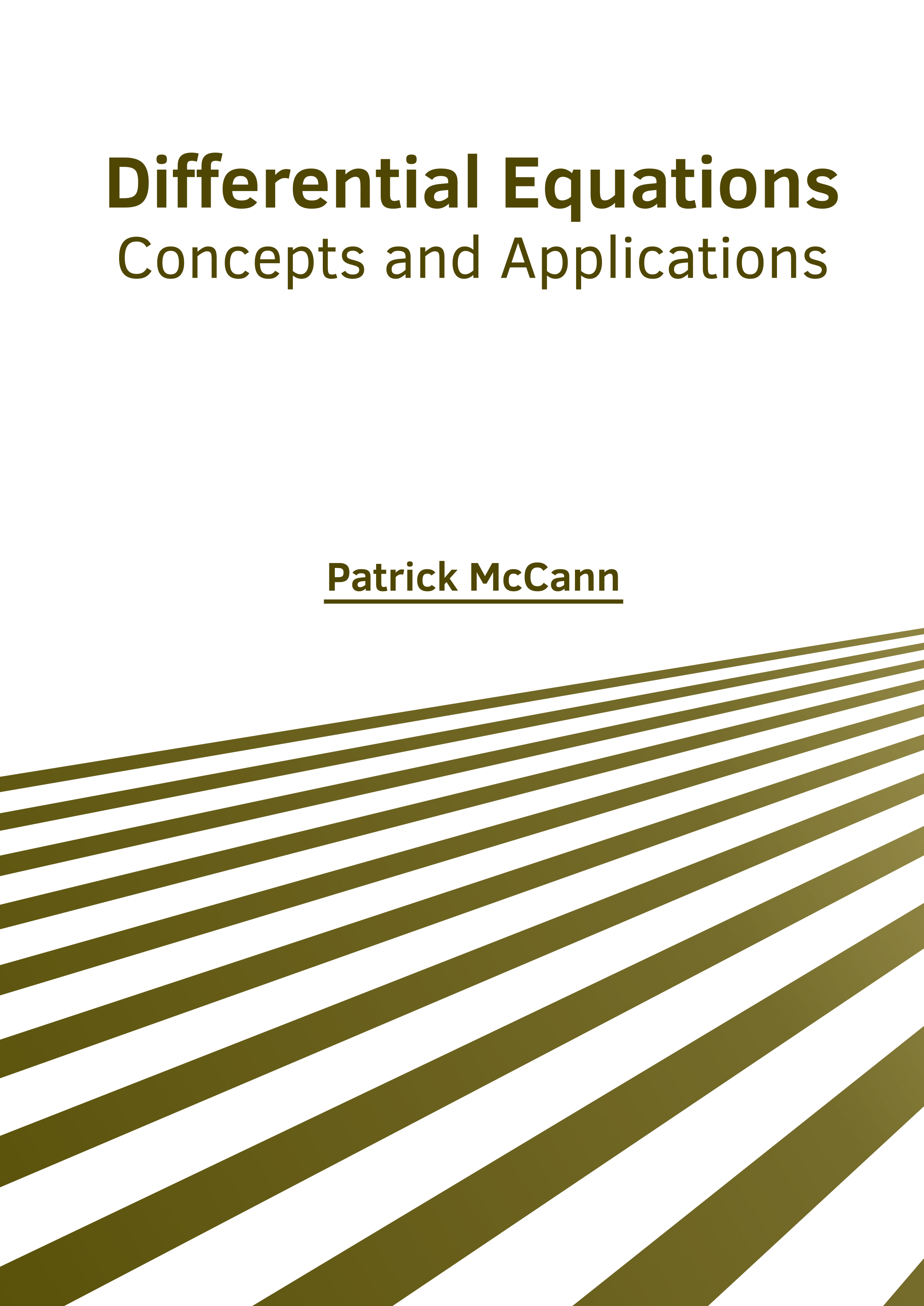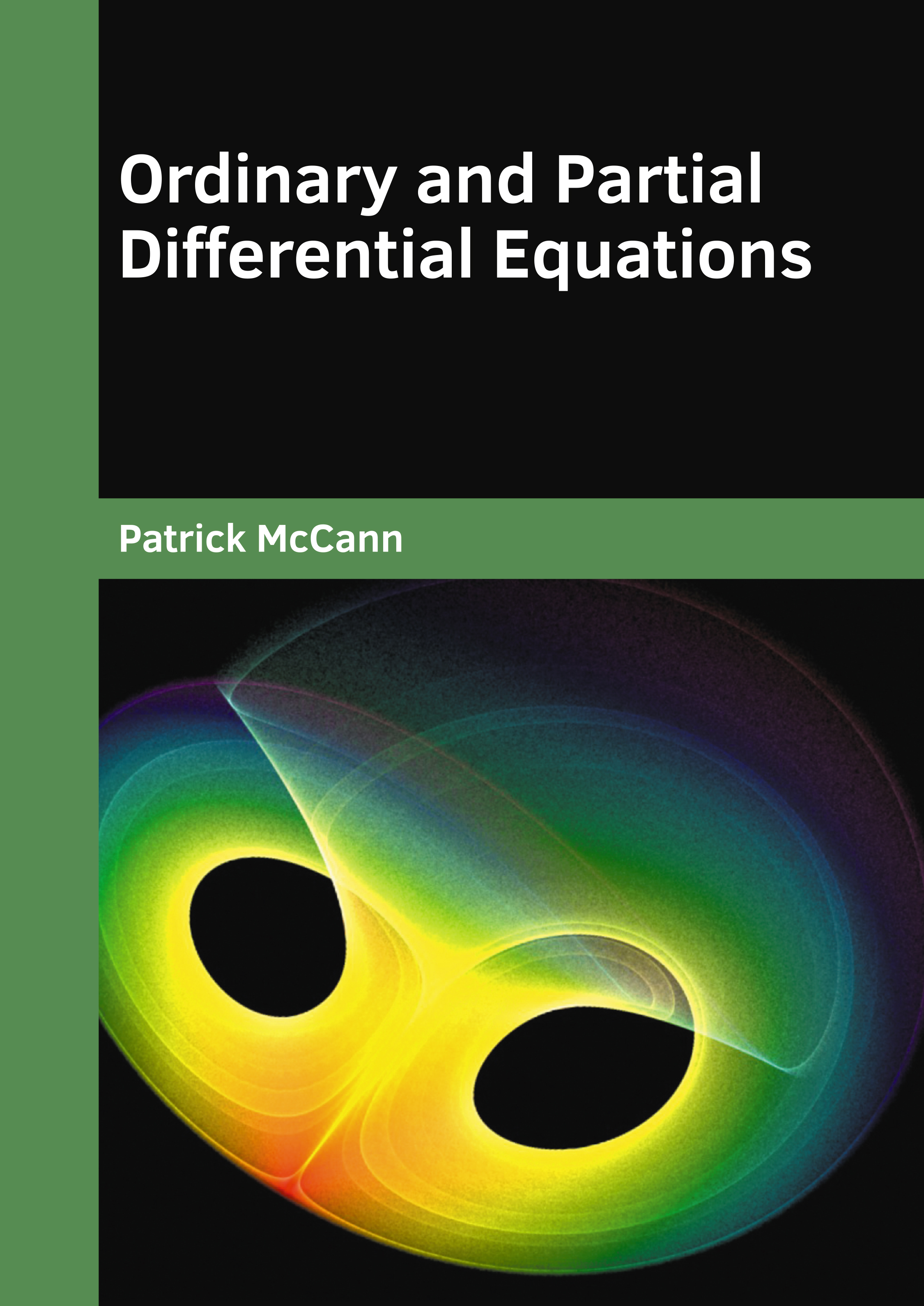BROWSE BY

#### Author

Results 1 - 2 of 2Differential Equations: Concepts and Applications Author : Patrick McCann Subject : Mathematics ISBN :9781632387622 Differential Equation is a mathematical equation which denotes the relationship between a function and its derivatives. The functions usually represent physical quantities in the applications and the derivatives Read MoreOrdinary and Partial Differential Equations Author : Patrick McCann Subject : Mathematics ISBN :9781682858240 The statement which expresses the equality of two expressions is known as an equation. A differential equation is a kind of mathematical equation that shows the connection between a function and its derivatives. Read More
Results 1 - 2 of 2